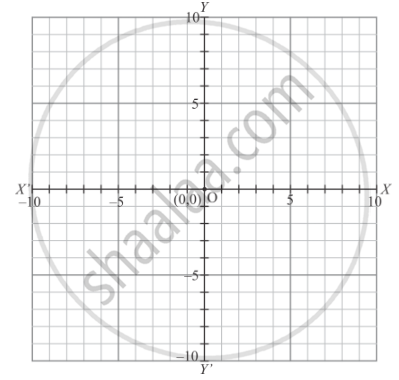# Plot the Following Point on the Graph Paper: (2,5) - Mathematics

Plot the following point on the graph paper:

(0, 0)

#### Solution

The following point is given below.

(0, 0)

Let X ' OX and  Y' OY be the coordinate axes.

Here for the given point the abscissa is 0 units and ordinate is 0 units. The point is basically intersection of the coordinate axes. So it will look like as shown in the following figure.Concept: Plotting a Point in the Plane If Its Coordinates Are Given.
Is there an error in this question or solution?

#### APPEARS IN

RD Sharma Mathematics for Class 9
Chapter 8 Co-ordinate Geometry
Exercise 8.1 | Q 1.1 | Page 6

Share